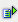The function count (range) calculates the number of empty cells within a range.

Syntax: =countblank(range)Example

Cell A5 = countblank(A1:A4)

 R / C A 1 1 2 text 3 4 3 5 1

The number of empty cells in the range A1 to A4 is 1